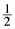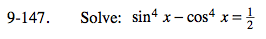### Home > PC > Chapter 9 > Lesson 9.3.4 > Problem9-147

9-147.

Solve: sin4 x − cos4 x =Homework Help ✎Factor.

Simplify using identity.

Substitute:

sin2x = 1 − cos2x

Simplify.

Divide both sides by −2.

Square root both sides.

Solve for x.

$(\text{sin}^2 x + \text{cos}^2 x)(\text{sin}^2 x - \text{cos}^2 x) = \frac{1}{2}$

$(\text{sin}^2 x - \text{cos}^2 x) = \frac{1}{2}$

$1-\text{cos}^2 x - \text{cos}^2 x = \frac{1}{2}$

$-2 \text{cos}^2 x = -\frac{1}{2}$

$\text{cos}^2 x = \frac{1}{4}$

$\text{cos} x = \pm \frac{1}{2 }$

$x = \pm \frac{\pi}{3}+\pi n$